Next: Projective Invariants & the Up: Linear Algebra and Homogeneous Previous: Lines in the Plane

## The Fixed Points of a Collineation

A point A is fixed by a collineation with matrix H exactly when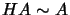, i.e.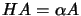for some scalar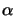. In other words, A must be a right eigenvector of H. Since an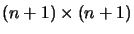matrix typically has n+1 distinct eigenvalues, a collineation in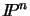typically has n+1 fixed points, although some of these may be complex.

H maps the line through any two fixed points A and B onto itself: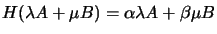. In addition, if A and B have the same eigenvalue (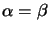),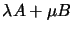is also an eigenvector and the entire line AB is pointwise invariant. In fact, the pointwise fixed subspaces ofunder H correspond exactly to the eigenspaces of H's repeated eigenvalues (if any).

Exercise 2.5   : Show that the matrix associated with a plane translation has a triple eigenvalue, but that the corresponding eigenspace is only two dimensional. Provide a geometric interpretation of this.

Exercise 2.6   : In 3D Euclidean space, consider a rotation by angle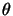about the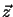axis. Find the eigenvalues and eigenspaces, prove algebraically that the rotation axis is pointwise invariant, and show that in addition the circular points'' with complex coordinates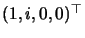and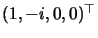are fixed.Next: Projective Invariants & the Up: Linear Algebra and Homogeneous Previous: Lines in the Plane
Bill Triggs
1998-11-13